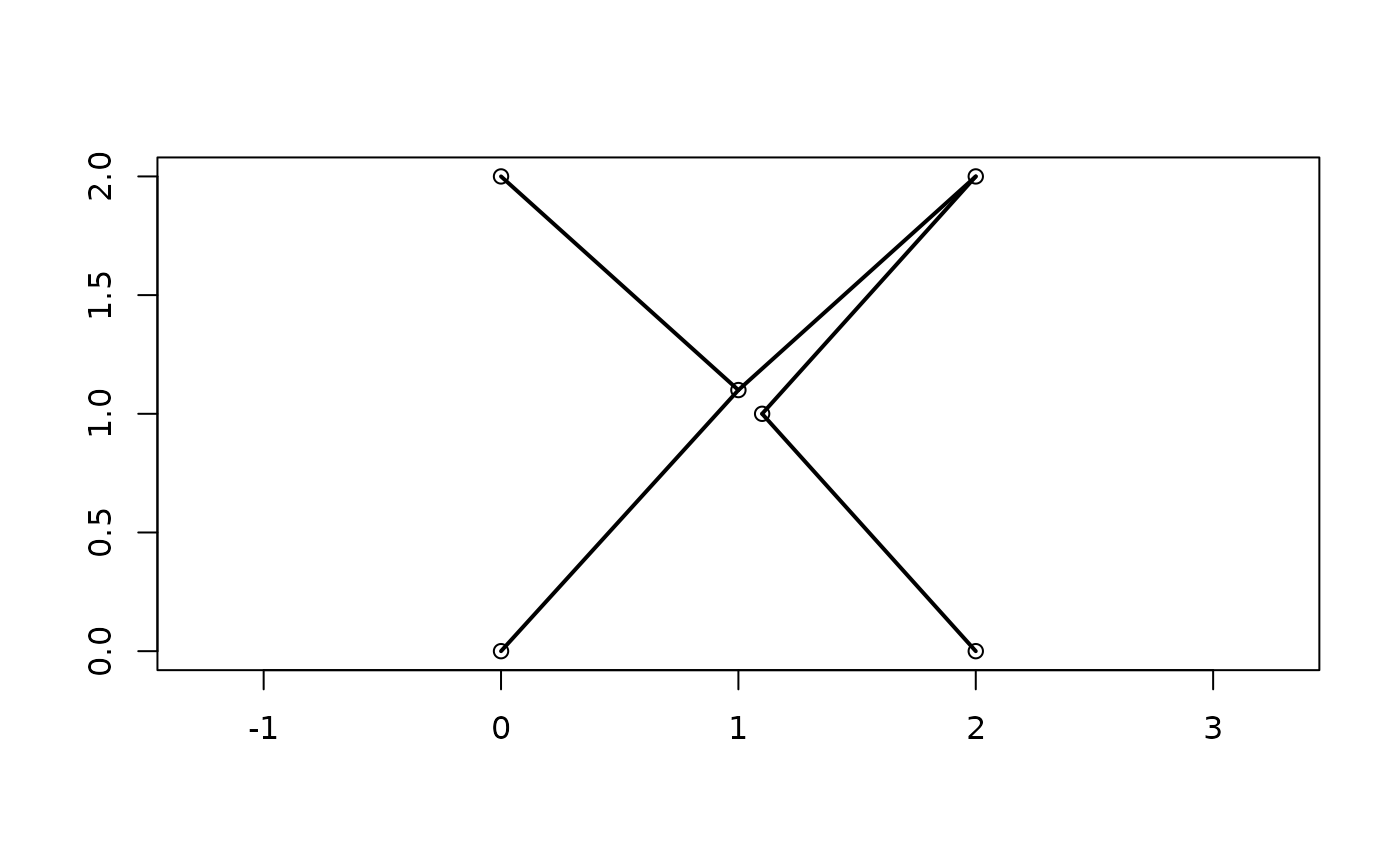Calculate or plot the minimum spanning tree (Gower and Ross 1969) of a distance matrix.

MSTEdges(distances, plot = FALSE, x = NULL, y = NULL, ...)

MSTLength(distances, mst = NULL)

## Arguments

distances

Either a matrix that can be interpreted as a distance matrix, or an object of class dist.

plot

Logical specifying whether to add the minimum spanning tree to an existing plot.

x, y

Numeric vectors specifying the X and Y coordinates of each element in distances. Necessary only if plot = TRUE.

...

Additional parameters to send to [lines()].

mst

Optional parameter specifying the minimum spanning tree in the format returned by MSTEdges(); if NULL, calculated from distances.

## Value

MSTEdges() returns a matrix in which each row corresponds to an edge of the minimum spanning tree, listed in non-decreasing order of length. The two columns contain the indices of the entries in distances that each edge connects, with the lower value listed first.

MSTLength() returns the length of the minimum spanning tree.

Slow implementation returning the association matrix of the minimum spanning tree: ape::mst().

## Examples

# Corners of an almost-regular octahedron
points <- matrix(c(0, 0, 2, 2, 1.1, 1,
0, 2, 0, 2, 1, 1.1,
0, 0, 0, 0, 1, -1), 6)
distances <- dist(points)
mst <- MSTEdges(distances)
MSTLength(distances, mst)
#>  8.496869
plot(points[, 1:2], ann = FALSE, asp = 1)
MSTEdges(distances, TRUE, x = points[, 1], y = points[, 2], lwd = 2)